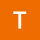Home Communities
IT Knowledge
Inspiration
Languages
EN

# Java - how to get element from HashSet?

6 points
Created by:Tehya-Blanchard
444

## 1. Overview

In java `Set` / `HashSet``LinkedHashSet` don't have get method.

Ofcourse it is possible to 'GET' element from Set in java, we just need to think a bit different when using this data structure. What I mean by this?

When we want to GET element from Set in java we just need to check if Set contains the object we want to get. So we already have our element we want to get from HashSet, the only think left to do is to check if Set have this object inside by using contains method with correctly implemented `hashCode() `and `equals()`.

HashSet internally uses HashMap and contains method on HashSet calls HashMap containsKey method.

Internally HashSet uses HashMap which looks like this:

``private transient HashMap<E,Object> map;``

We can go inside JDK and check it by ourselves.

The best way to understand what I mean is by analyzing below 2 examples.

When we use intellij IDEA we can just click on contains method and attach the debugger inside.

## 2. Example 1 - HashSet with String

This example uses String, String have internal hashCode and equals implemented in JDK.

``````import java.util.HashSet;
import java.util.Set;

public class JavaGetElementFromHashSetExample1 {

public static void main(String[] args) {

Set<String> set = new HashSet<>();

// get A
// we already have String "A"
// so we just only need to check if "A" exists in HashSet
System.out.println(set.contains("A")); // true

// get D
System.out.println(set.contains("D")); // false
}
}``````

Output:

``````true
false``````

String - Internal hashCode and equals implemented in JDK.

``````public int hashCode() {
int h = hash;
if (h == 0 && value.length > 0) {
char val[] = value;

for (int i = 0; i < value.length; i++) {
h = 31 * h + val[i];
}
hash = h;
}
return h;
}

public boolean equals(Object anObject) {
if (this == anObject) {
return true;
}
if (anObject instanceof String) {
String anotherString = (String)anObject;
int n = value.length;
if (n == anotherString.value.length) {
char v1[] = value;
char v2[] = anotherString.value;
int i = 0;
while (n-- != 0) {
if (v1[i] != v2[i])
return false;
i++;
}
return true;
}
}
return false;
}``````

HashSet - contains JDK implementation (as we can see HashSet internally uses HashMap)

``````package java.util;

public class HashSet<E>
extends AbstractSet<E>
implements Set<E>, Cloneable, java.io.Serializable
{
// ...

private transient HashMap<E,Object> map;

// ...

public boolean contains(Object o) {
return map.containsKey(o);
}

// ...
}``````

## 3. Example 2 - HashSet with custom User objects

In this example we implement explicit hashCode and equals for User class.

When we invoke contains method

``````import java.util.HashSet;
import java.util.Objects;
import java.util.Set;

public class JavaGetElementFromHashSetExample2 {

public static void main(String[] args) {

Set<User> set = new HashSet<>();

// get 1st user by checking if set contains our User object
// User class implement custom hash code and equals method
// we use key User(1L, "A") to 'get' user from Set
// we already have this object so we just only need to check if
// object is in our hash set
System.out.println(set.contains(new User(1L, "A"))); // true

// get 4th user
System.out.println(set.contains(new User(4L, "D"))); // false
}

private static class User {
private long userId;

public User() {

}

public User(long userId, String username) {
this.userId = userId;
}

public long getUserId() {
return userId;
}

public void setUserId(long userId) {
this.userId = userId;
}

}

}

@Override
public boolean equals(Object o) {
if (this == o) return true;
if (o == null || getClass() != o.getClass()) return false;
User user = (User) o;
return userId == user.userId &&
}

@Override
public int hashCode() {
}

@Override
public String toString() {
return "User{" +
"userId=" + userId +
'}';
}
}
}``````

Output:

``````true
false``````

Join to our subscribers to be up to date with content, news and offers.